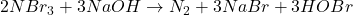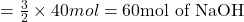## 1. Consider the chemical equation. If there are 40 mol of NBr3 and 48 mol of NaOH, what is the limiting reactant? 2NBr3 + 3NaOH –

Question

1. Consider the chemical equation. If there are 40 mol of NBr3 and 48 mol
of NaOH, what is the limiting reactant?
2NBr3 + 3NaOH –> N2 + 3NaBr + 3HOBI
ON2
ONBr3
O NaOH
O HOBI​

in progress 0
5 months 2021-08-09T16:29:36+00:00 1 Answers 39 views 0

The correct answer is the third option.

Explanation:Moles of nitrogen tribromide = 40 mol

Moles of sodium hydroxide = 48 mol

According to reaction, 2 moles of nitrogen tribromide reacts with 3 moles of sodium hydroxide, then 40 mol of nitrogen tribromide will react with:This means that in order to completely react with 40 moles of nitrogen tribromide we will need 60 moles of sodium hydroxide.

But according to the question we only have 48 moles of sodium hydroxide which is less than the 60 moles of sodium hydroxide which indicates that sodium hydroxide is present in a limited amount and nitrogen tribromide is present in an excess amount.

So, the limiting reagent is sodium hydroxide, hence the correct answer is the third option.